Cisco CCENT  Subnetting Part II – Math to Memorize!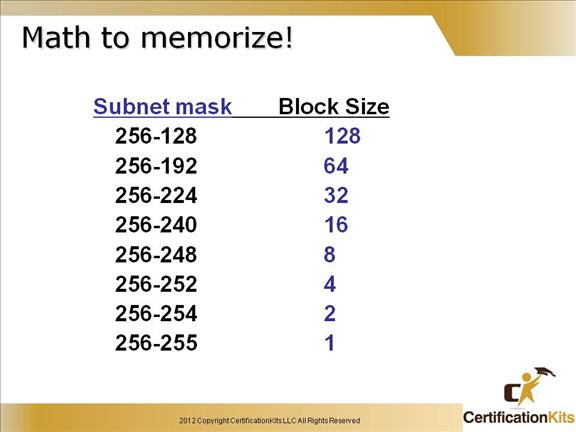Block sizes will be discussed in more detail later.

Cisco CCENT Powers of 2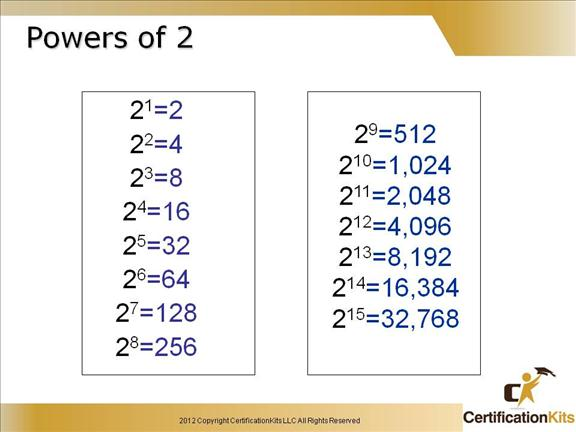Powers of 2 are important to understand and memorize for use with IP subnetting.

To review powers of 2, remember that when you see a number with another number to its upper right (called an exponent), this means you should multiply the number by itself as many times as the upper number specifies.

For example, 23 is 2 ´ 2 ´ 2, which equals 8.

Cisco CCENT What do you know?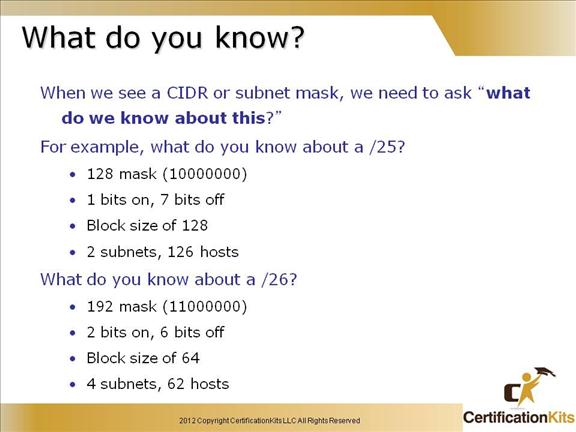This slide and the next few slides are added for your studying enjoyment and to help you memorize important class C subnetting information.

If you can remember this information, subnetting will be a bread

Cisco CCENT What do we know?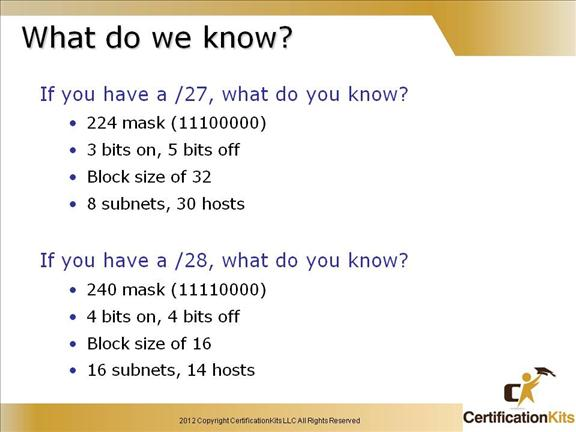Cisco CCENT What do we know?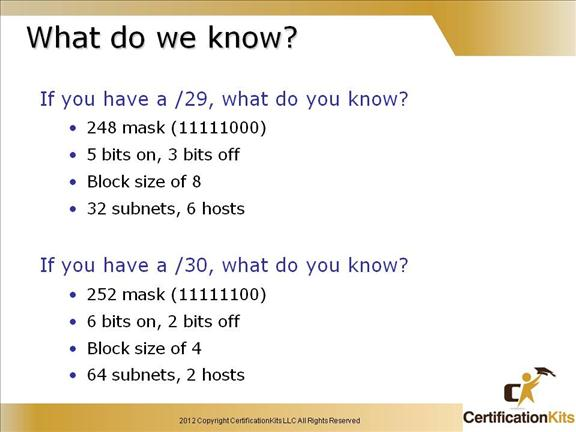Cisco CCENT Easy Subnetting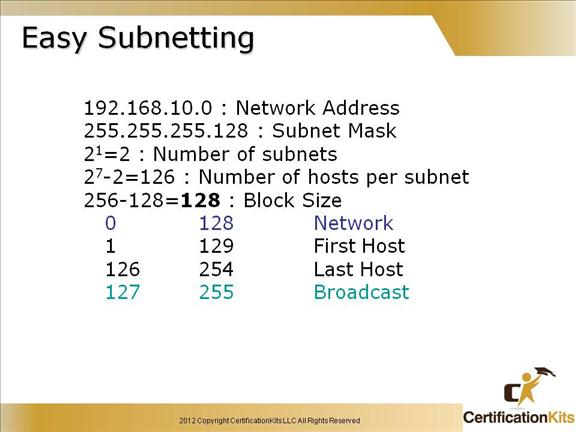How many subnets?

Since 128 is one bit on (10000000), the answer would be 21 = 2.

How many hosts per subnet? We have 7 host bits off (10000000), so the equation would be 27 – 2 = 126 hosts.

What are the valid subnets? 256 – 128 = 128, which our block size.  Start with 0 and keep adding the block size to get our valid subnets.

Our valid subnets are, then, 0 and 128.

Whats the broadcast address for each subnet? The number right before the next subnet is all host bits turned on and equals the broadcast address.

What are the valid hosts? These are the numbers between the subnets. The easiest way to find the hosts is to write out the subnet address and the broadcast address. This way the valid hosts are obvious.

Cisco CCENT Now, implement it…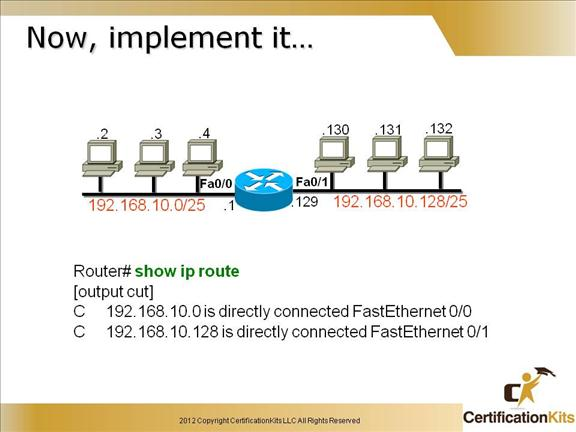This slide shows the output of a “show ip route” command when a Class C network is subnetted to a /25 (255.255.255.128) mask.

The routers can use any valid host address in the subnet, the hosts just need to know what it is so they can set the default gateway to that address.

The slide provides basic information on how you would implement a /25 Class C network and the routers routing table.  Each subnet supports 126 addresses per subnet, 128 total addresses (equals block size)  where the first address is for the network and the last address is the broadcast address.

Cisco CCENT Easy Subnetting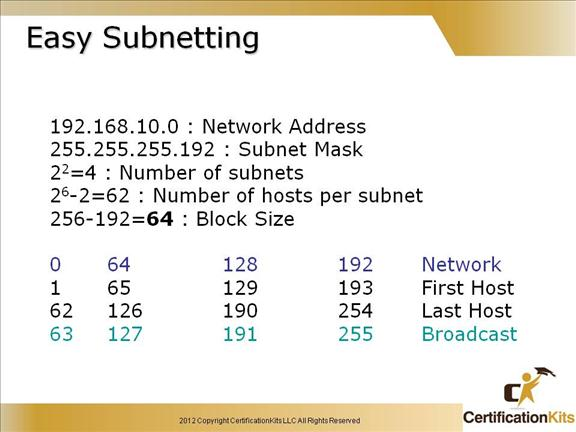How many subnets?

Since 192 is two bits on (11000000), the answer would be 22 = 4.

How many hosts per subnet? We have 6 host bits off (11000000), so the equation would be 26 – 2 = 62 hosts.

What are the valid subnets? 256 – 192 = 64, which gives us our block size.  Start with 0 and keep adding the block size to get our valid subnets.

Our valid subnets are, then, 0, 64, 128 and 192.

Whats the broadcast address for each subnet? The number right before the next subnet is all host bits turned on and equals the broadcast address.

What are the valid hosts? These are the numbers between the subnets and the broadcast address. The easiest way to find the hosts is to write out the subnet address and the broadcast address. This way the valid hosts are obvious.

Cisco CCENT Now, implement it…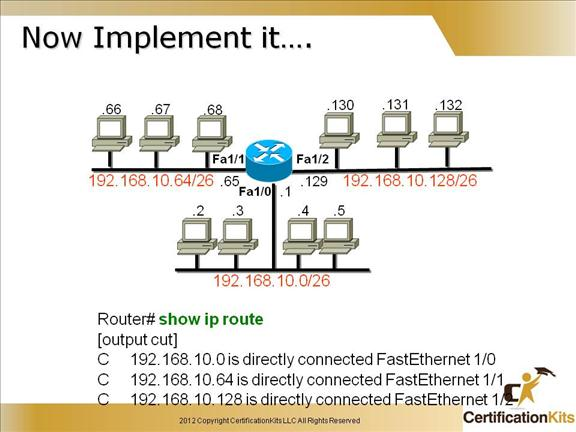This slide shows the output of a “show ip route” command when a Class C network is subnetted to a /26 (255.255.255.192) mask.

The routers can use any valid host address in the subnet, the hosts just need to know what it is so they can set the default gateway to that address. Each subnet supports 62 addresses per subnet, 64 total addresses (equals block size) where the first address is for the network and the last address is the broadcast address.

Cisco CCENT Easy Subnetting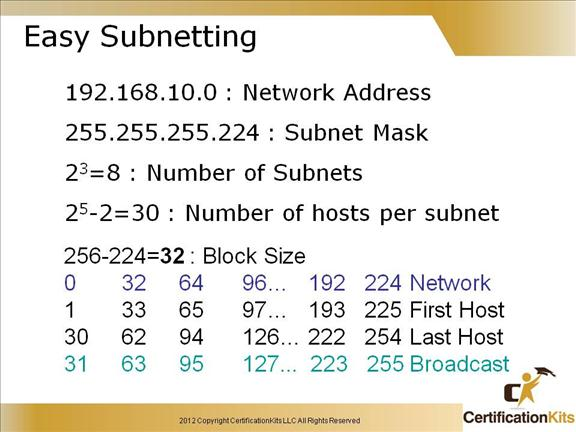How many subnets? 224 is 11100000, so our equation would be 23 = 8.

How many hosts? 25 – 2 = 30.

What are the valid subnets? 256 – 224 = 32. 32 + 32 = 64. 64 + 32 = 96. 96 + 32 = 128. 128 + 32 = 160. 160 + 32 = 192. 192 + 32 = 224, which is invalid because it is our subnet mask (all subnet bits on). Our subnets are 0, 32, 64, 96, 128, 160, 192 and 224.

Whats the broadcast address for each subnet? Always the number right before the next subnet

What are the valid hosts? The numbers between the subnet number and the broadcast address

Cisco CCENT Now, implement it…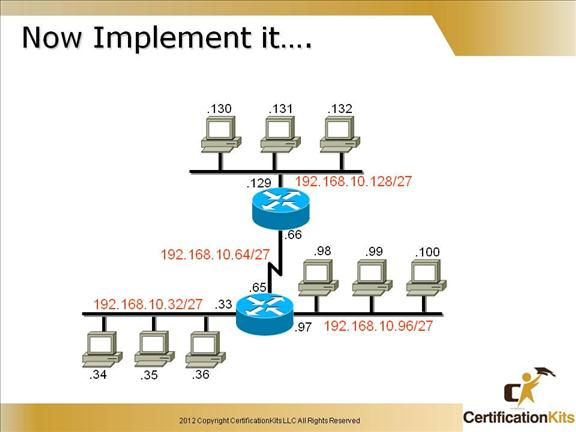This slide shows the output of a “show ip route” command when a Class C network is subnetted to a /27 (255.255.255.224) mask.

The routers can use any valid host address in the subnet, the hosts just need to know what it is so they can set the default gateway to that address.

Cisco CCENT Easy Subnetting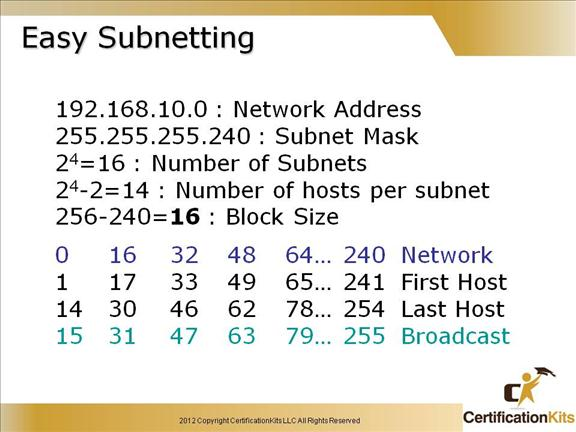Subnets? 240 is 11110000 in binary. 24 = 16.

Hosts? Four host bits, or 24 – 2 = 14.

Valid subnets? 256 – 240 = 16. 16 + 16 = 32. 32 + 16 = 48. 48 + 16 = 64. 64 + 16 = 80. 80 + 16 = 96. 96 + 16 = 112. 112 + 16 = 128. 128 + 16 = 144. 144 + 16 = 160. 160 + 16 = 176. 176 + 16 = 192. 192 + 16 = 208. 208 + 16 = 224. 224 + 16 = 240. So, our valid subnets are 0, 16, 32, 48, 64, 80, 96, 112, 128, 144, 160, 176, 192, 208, 224and 240.

Valid hosts?

Cisco CCENT Easy Subnetting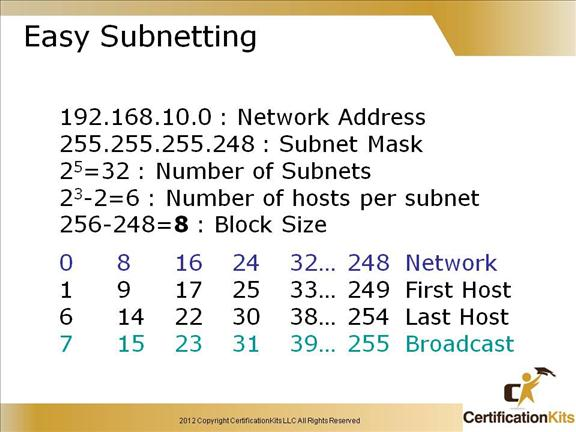Subnets? 248 in binary = 11111000. 25 = 32.

Hosts? 23 –2 = 6.

Valid subnets? 256 – 248 = 0, 8, 16, 24, 32, 40, 48, 56, 64, 72, 80, 88, 96, 104, 112, 120, 128, 136, 144, 152, 160, 168, 176, 184, 192, 200, 208, 216, 224, 232, 240, and 248.

Valid hosts?

Cisco CCENT Easy Subnetting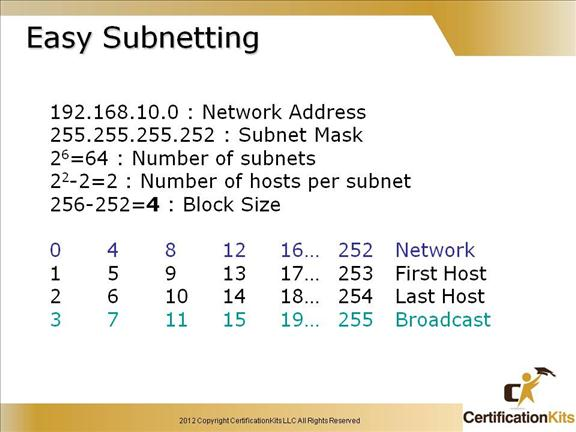Subnets? 64.

Hosts? 2.

Valid subnets? 256 – 252 = 4 which gives us our block size. Start with 0 and keep adding the block size to get our  valid subnets.

0, 4, 8, 12, etc., all the way to 252.

Broadcast address for each subnet? Always the number right before the next subnet.

Valid hosts? The numbers between the subnet number and the broadcast address.

Cisco CCENT Now, implement it…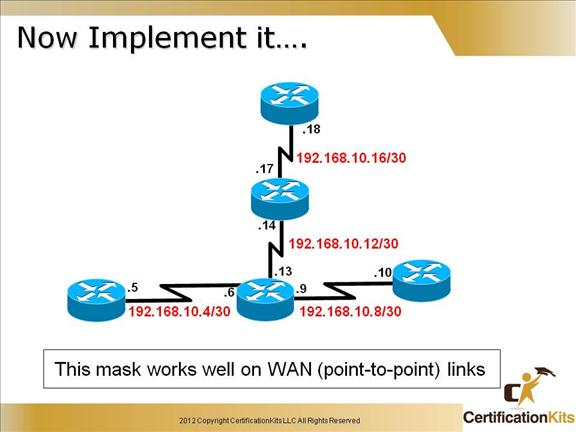It is very common to use 255.255.255.252 or /30 bit mask on point-to-point links.  Although you can use a larger range, since there is only two possible hosts on a point-to-point link, it makes sense to assign a /30 network to it.  Doing this we can save on valuable IP addresses. Each subnet supports 2 addresses per subnet, 4 total addresses (equals block size) where the first address is for the network and the last address is the broadcast address.

Cisco CCENT Easy Subnetting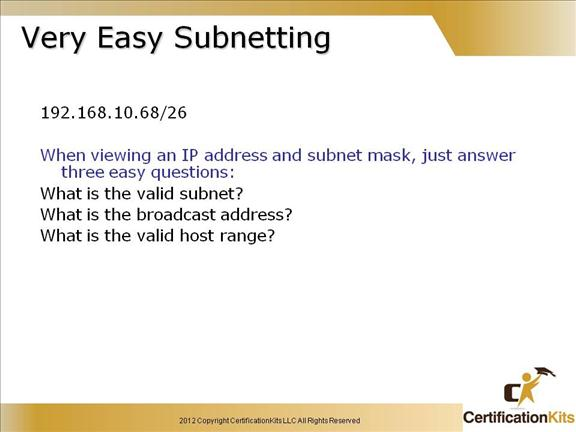256-192=       0          64        128     Subnet

1          65        129     First Host

62        126     190     Last Host

There is another subnet available (192), but when we are discussing very easy subnetting, we are just trying to find the subnet that the host address is a member of.

Cisco CCENTUnicast / Broadcast / Multicast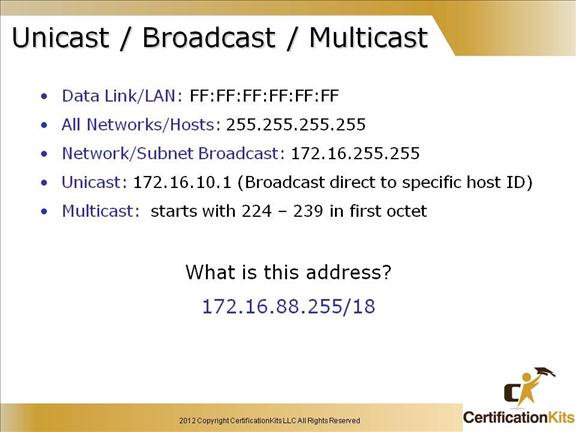It is important to understand the different terms and uses of broadcast addresses.

There are four different types:

üLayer 2 broadcasts: broadcast that is sent to all nodes on a LAN

üBroadcasts (layer 3): broadcast that is sent to all nodes on the network

üUnicast: broadcast sent to a single destination host

üMulticast: broadcast that is sent from a single source and transmitted to many devices on different networks